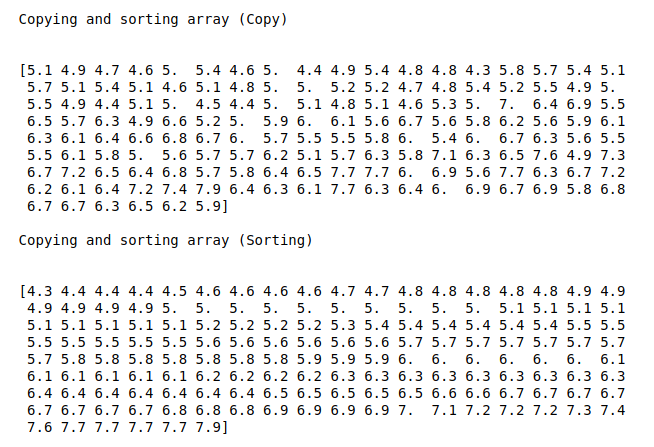• #5, First Floor, 4th Street Dr. Subbarayan Nagar Kodambakkam, Chennai-600 024 Landmark : Samiyar Madam
• pro@slogix.in
• +91- 81240 01111

### How to perform arithmetic operations in an array using numpy?

###### Description

To write a piece of python code to perform arithmetic operations of Numpy array.

#### Input

Array of data. (Iris data)

#### Output

Arithmetic operations results.

###### Process

Create two array.

Do arithmetic operations of an array using Numpy.

Print the results.

###### Sample Code

#import libraries
import pandas as pd
import numpy as np

#load the sample data from csv file

#convert as a data frame
df = pd.DataFrame(data)

a = np.array(df.iloc[:,0])
b = np.array(df.iloc[:,1])

print(“First array\n\n”,a,”\n”)
print(“Second array\n\n”,b,”\n”)

#arithmetic operations
print(“\n”)

print(“Aritmetic operations results (subtraction)\n\n”)
print(np.subtract(a,b))
print(“\n”)

print(“Aritmetic operations results (Division)\n\n”)
print(np.divide(a,b))
print(“\n”)

#Comparison operations
print(“logical operations (comparison)\n\n”)
print(a != b)
print(“\n”)
print(a>=6,”\n”)

#aggregate functions
print(“Aggregete functions (sum)”)
print(np.sum(a),”\n”)

print(“Aggregete functions (minimum)”)
print(np.min(a),”\n”)

print(“Aggregete functions (mean)”)
print(np.mean(a),”\n”)

#Copying and sorting arrays
print(“Copying and sorting array (Copy)\n\n”)
print(np.copy(a),”\n”)

print(“Copying and sorting array (Sorting)\n\n”)
print(np.sort(a),”\n”)

###### Screenshots